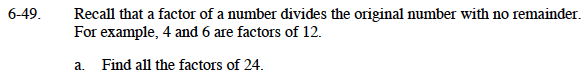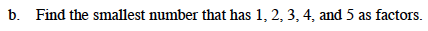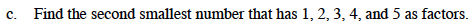### Home > MC1 > Chapter 6 > Lesson 6.2.1 > Problem6-49

6-49.
1. . Recall that a factor of a number divides the original number with no remainder. For example, 4 and 6 are factors of 12. Homework Help ✎

1. Find all the factors of 24.

2. Find the smallest number that has 1, 2, 3, 4, and 5 as factors.

3. Find the second smallest number that has 1, 2, 3, 4, and 5 as factors.Remember that factors go together.
For example, if 12 goes into 24 exactly twice, then 2 must go into 24 exactly 12 times.